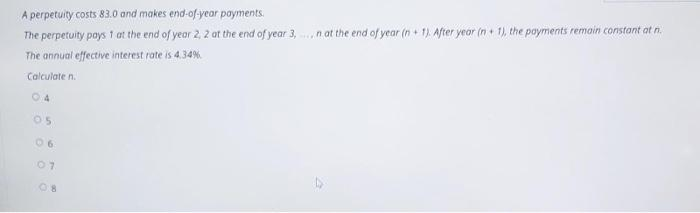Home / Expert Answers / Finance / a-perpetuity-costs-83-0-and-makes-end-of-year-poyments-the-perpetuity-pays-1-at-the-end-of-ye-pa335

# (Solved): A perpetuity costs $$83.0$$ and makes end-of-year poyments The perpetuity pays 1 at the end of ye ...A perpetuity costs $$83.0$$ and makes end-of-year poyments The perpetuity pays 1 at the end of year 2,2 of the end of year $$3, \ldots, n$$ at the end of year $$(n+1)$$. Atrer year $$(n+1)$$, the poyments cemain constant at $$n$$. The annual effective interest rate is $$4.34 \%$$. Calculate $$\mathrm{n}$$. 4 5 16. 7 8

We have an Answer from Expert